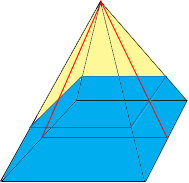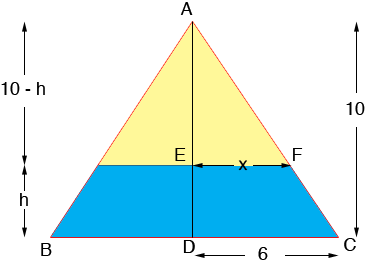SEARCH HOMEMath Central Quandaries & QueriesQuestion from andrew, a student: Hi, I've been having real trouble visualizing this problem as apposed to a conical tank. It says the base of a pyramid-shaped tank is a square with sides of length 12 feet. The vertex of the pyramid is 10 feet above the base. The tank is filled to a depth of 4 feet, water is flowing into the tank at the rate of 2 cubic feet per minute. Find the rate of change of the depth of water in the tank.Hi Andrew,

I drew a diagram of the tank to make sure we are talking about the same situation. A fact I am going to need is that the volume of a pyramid is

1/3 × the area of the base × the height.Now I want to slice through the tank through the plane containing the red lines and look at the cross section.The height of the water is h feet and the top surface of the water is a square that is 2x feet on each side. Since water is flowing into the tank both h and x are functions of time. The volume V of the water in the tank is also a function of time and you know that dV/dt = 2 cu ft/min where t is in minutes. Your task is to find dh/dt at a specific time. To do this you are going to need an expression for V, the volume of water in the tank.

The volume of the tank is 1/3 122 × 10 cubic feet and hence you can find V by subtracting the volume of the empty portion of the tank, the piece shaded yellow in the diagram. This also is a pyramid and its volume is

1/3 (2x)2 × (10 - h)

Hence you have an expression for V but it is a function of two variables x and h. You can use the fact that triangles ADC and AEF are similar to find a relationship between x and h and hence eliminate x from the expression for V.

I hope this helps,
HarleyMath Central is supported by the University of Regina and The Pacific Institute for the Mathematical Sciences.